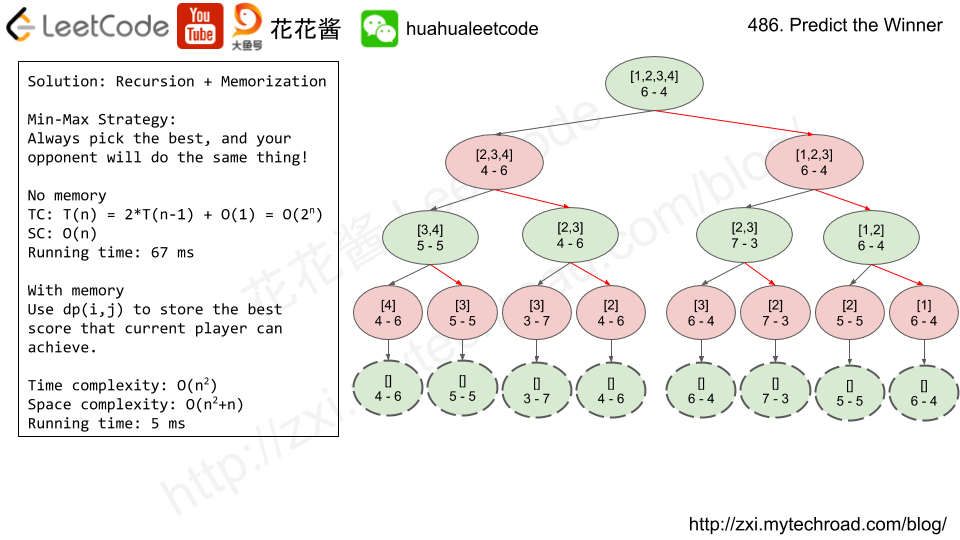Press "Enter" to skip to content

# Problem

Given an array of scores that are non-negative integers. Player 1 picks one of the numbers from either end of the array followed by the player 2 and then player 1 and so on. Each time a player picks a number, that number will not be available for the next player. This continues until all the scores have been chosen. The player with the maximum score wins.

Given an array of scores, predict whether player 1 is the winner. You can assume each player plays to maximize his score.

Example 1:
Input: [1, 5, 2]
Output: False
Explanation: Initially, player 1 can choose between 1 and 2.
If he chooses 2 (or 1), then player 2 can choose from 1 (or 2) and 5. If player 2 chooses 5, then player 1 will be left with 1 (or 2).
So, final score of player 1 is 1 + 2 = 3, and player 2 is 5.
Hence, player 1 will never be the winner and you need to return False.

Example 2:
Input: [1, 5, 233, 7]
Output: True
Explanation: Player 1 first chooses 1. Then player 2 have to choose between 5 and 7. No matter which number player 2 choose, player 1 can choose 233.
Finally, player 1 has more score (234) than player 2 (12), so you need to return True representing player1 can win.
Note:
1 <= length of the array <= 20.
Any scores in the given array are non-negative integers and will not exceed 10,000,000.
If the scores of both players are equal, then player 1 is still the winner.# Solution 1: Recursion

Time complexity: O(2^n)

Space complexity: O(n)

# Solution 2: Recursion + Memoization

Time complexity: O(n^2)

Space complexity: O(n)

DP version

# Related Problem

Given a string, sort it in decreasing order based on the frequency of characters.

Example 1:

Example 2:

Example 3:

Solution:

Given a word, you need to judge whether the usage of capitals in it is right or not.

We define the usage of capitals in a word to be right when one of the following cases holds:

1. All letters in this word are capitals, like “USA”.
2. All letters in this word are not capitals, like “leetcode”.
3. Only the first letter in this word is capital if it has more than one letter, like “Google”.

Otherwise, we define that this word doesn’t use capitals in a right way.

Example 1:

Example 2:

Note: The input will be a non-empty word consisting of uppercase and lowercase latin letters.

Given an array of integers, 1 ≤ a[i] ≤ n (n = size of array), some elements appear twice and others appear once.

Find all the elements that appear twice in this array.

Could you do it without extra space and in O(n) runtime?

Example:

Given an unsorted array of integers, find the length of the longest consecutive elements sequence.

For example,
Given [100, 4, 200, 1, 3, 2],
The longest consecutive elements sequence is [1, 2, 3, 4]. Return its length: 4.

Your algorithm should run in O(n) complexity.

Mission News Theme by Compete Themes.